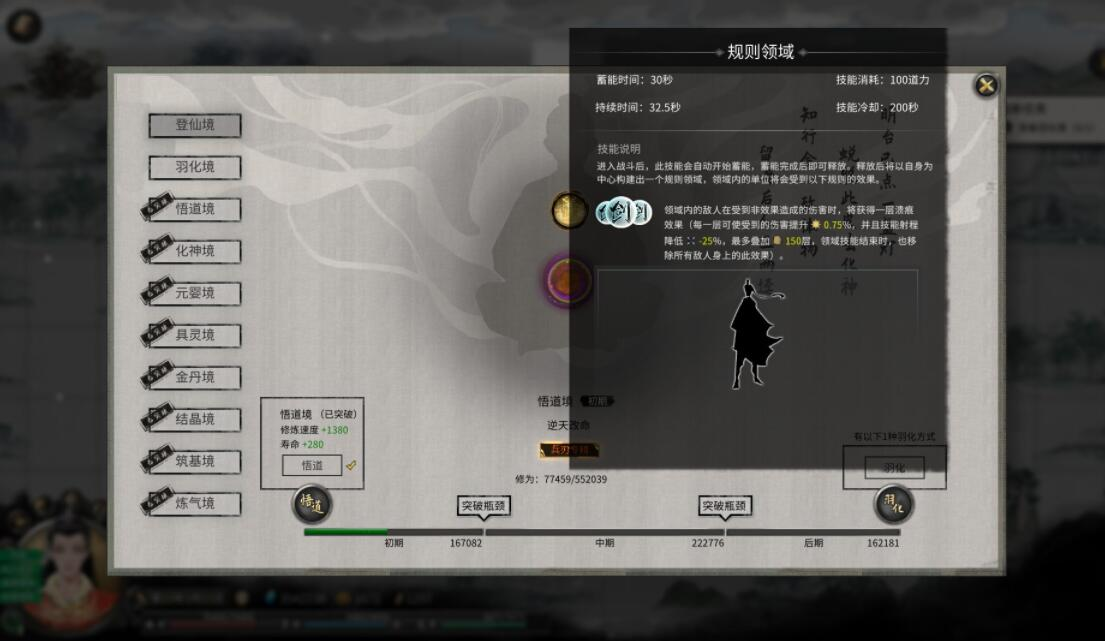# 鬼谷八荒道力值怎么恢复## 鬼谷八荒道力值恢复计算方法介绍：### 道力值上限计算公式

1+2+3=180

1+1+2=150

1+1+3=150

2+2+1=150

2+2+3=210

3+3+1=210

3+3+2=240

#### 相关专题#### 修仙游戏推荐